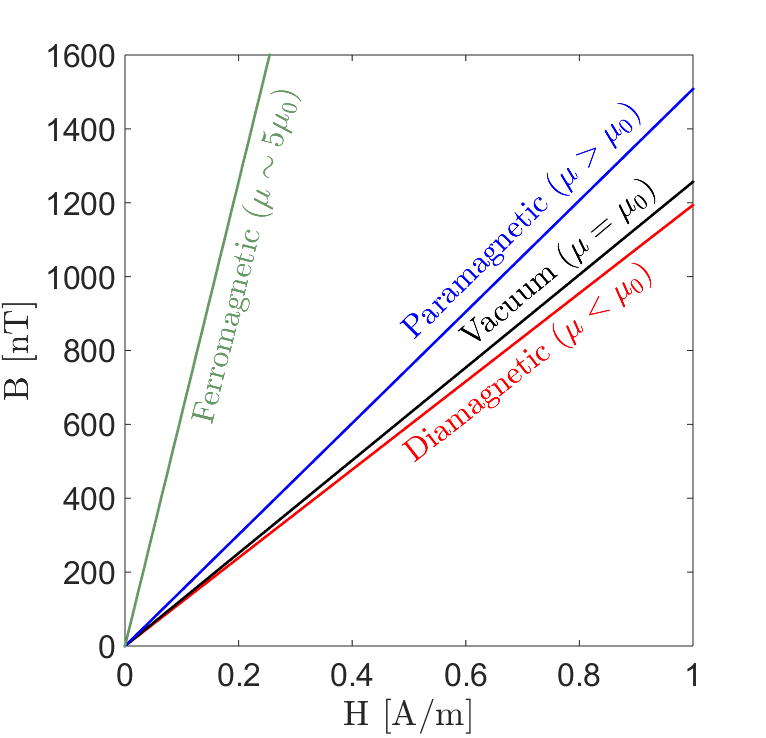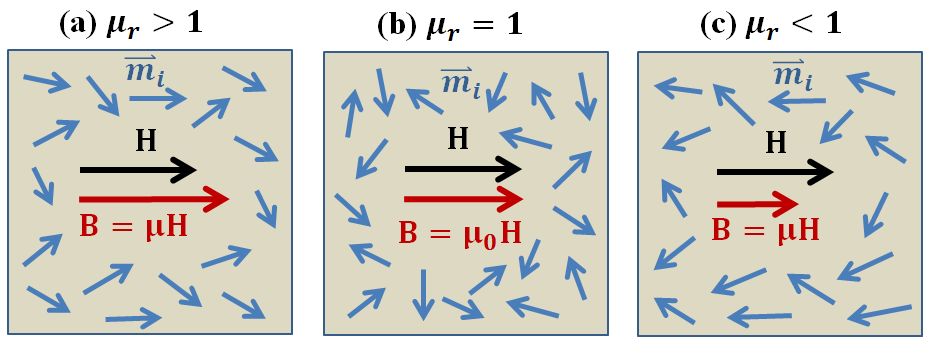# Magnetic Permeability

Magnetic permeability is a diagnostic physical property which characterizes the degree of induced magnetism a material experiences under the influence of an external magnetic field. Magnetic permeability is relevant to a variety of geophysical surveys, including: frequency-domain EM (FDEM), time-domain EM (TDEM), unexploded ordnance (UXO) and ground penetrating radar (GPR).

## Constitutive Relationship

When exposed to an applied magnetic field, the collection of individual magnetic dipole moments within most materials will attempt to reorient themselves along the direction of the field. This generates an induced magnetization, which contributes towards the net magnetic flux density inside the material. The degree in which the induced magnetization impacts the magnetic flux density depends on the material’s magnetic permeability.Fig. 25 Magnetic flux density as a function of magnetic field intensity for various classifications of permeable rock types: diamagnetic, vacuum, paramagnetic, and ferromagnetic.

Magnetic permeability $$\mu$$ defines the ratio between the magnetic flux density $${\bf B}$$ within a material, and the intensity of an applied magnetic field $${\bf H}$$; provided the fields are sufficiently weak:

(10)${\bf B}(\omega) = \mu \, {\bf H}(\omega)$

In a vacuum, the relationship between $${\bf B}$$ and $${\bf H}$$ is given by the permeability of free-space $$\mu_0 = 4\pi \times 10^{-7}$$ H/m. An illustration representing the magnetic flux density as a function of the applied field strength for various rock classifications is shown here.

## Relative Permeability

In addition to the magnetic permeability, magnetic properties are frequently represented using the relative permeability. Relative permeability characterizes whether the induced magnetization increases or reduces the density of magnetic flux within a material. The relative permeability $$\mu_r$$ is the ratio between the magnetic permeability of a material and the permeability of free-space:

(11)$\mu_r = \frac{\mu}{\mu_0}$

For the majority of rocks, induced magnetization is parallel to the applied field, thus adding to the density of magnetic flux. These rocks are characterized by relative permeabilities $$\mu_r > 1$$. A relative permeability of $$\mu_r = 1$$ is used to characterize materials which are incapable of supporting induced magnetization. In rare cases, a very small magnetization can be induced in rocks, which opposes the applied field, and reduces the density of magnetic flux. These rocks are characterized by magnetic permeabilities $$\mu_r < 1$$. The partial alignment of magnetic dipole moments under an applied field, and the resulting magnetic flux densities in all three cases, are represented in the figure below.Fig. 26 Partial alignment of magnetic dipole moments under the influence of an applied magnetic field for various cases. (a) Paramagnetic ($$\mu_r > 1$$). Magnetization is parallel to the applied field and increases the density of magnetic flux. (b) Non-permeable ($$\mu_r = 1$$). Does not support induced magnetization. (c) Diamagnetic ($$\mu_r < 1$$). Magnetization is weak and opposes the applied magnetic field, thus reducing the density of magnetic flux.

## Importance to Geophysics

Inductive Source EM Systems

In most geological environments, variations in the Earth’s magnetic permeability are insignificant ($$\mu \sim \mu_0$$) and surveys are only sensitive to contrasts in the Earth’s electrical conductivity. However, there are a variety of cases where magnetic permeability becomes an important diagnostic physical property. For example, some ore-bearing rocks (magnetite, pyrrhotite) have very high magnetic permeabilities. When using frequency-domain (FDEM) and time-domain (TDEM) electromagnetic systems, large magnetic permeabilities have been shown to impact the measured response. Thus failure to account for the Earth’s magnetic properties can result in a mis-characterization of subsurface structures in certain environments.

Unexploded Ordnance Surveys

Magnetic permeability is also a diagnostic physical property for unexploded ordnance (UXO) surveys. An unexploded ordnance (UXO) is a munition that was armed, fired and remains unexploded through malfunction. UXO casings are extremely permeable in comparison to the host medium. This contrast can be exploited in order to locate an remove UXOs from contaminated areas. The UXO’s magnetic properties are also responsible for producing distinct TDEM responses.